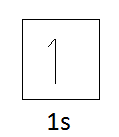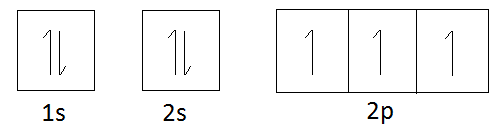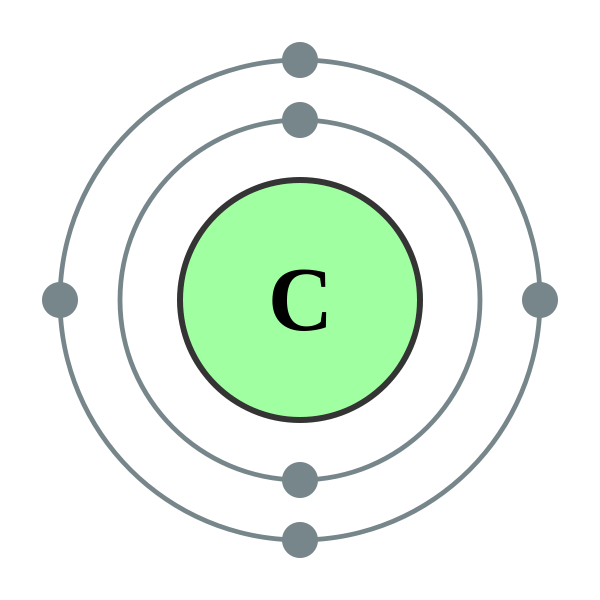# Difference Between Valence and Valency

## Main Difference – Valence vs Valency

Valence and valency are described in relation to the electrons present in an atom. Electrons are known to be located in shells or orbitals around the nucleus. Therefore, electrons can be lost from an atom according to the strength of the attraction force between these electrons and the nucleus of an atom. The valence and valency are related to the electrons in the outermost orbital of an atom. The main difference between valence and valency is that valence refers to the ability of an atom to be combined with another atom whereas valency refers to the maximum number of electrons that an atom can lose or gain in order to stabilize itself.

### Key Areas Covered

1. What is Valence
– Definition, Explanation with Examples
2. What is Valency
– Definition, Explanation with Examples
3. What are the Similarities Between Valence and Valency
– Outline of Common Features
4. What is the Difference Between Valence and Valency
– Comparison of Key Differences

Key Terms: Atom, Carbon Atom, Electrons, Hydrogen Atom, Nitrogen, Octet Rule, Orbital Diagram, Valence, Valence Electrons, Valency## What is Valence

Valence refers to the ability of an atom to be combined with another atom. In other words, it is the degree of combining strength of a particular atom. The valence of an atom is related to the number of electrons present in the outermost orbital of an atom. These electrons are called valence electrons. In order to be combined with another atom, a particular atom should have valence electrons that can be either lost, gained or can be paired. This is because a combination of two atoms can be both through an ionic bond or covalent bond, and in both these types, the atoms lose electrons, gain electrons or share electrons.

The valence of an atom can be given as the number of univalent atoms that can be attached to that atom or the number of single bonds that an atom can have. This can also be defined as the number of Hydrogen atoms (H) that can be attached to an atom. This is because Hydrogen atoms are univalent and can easily bind with other atoms. Some atoms can be bonded to other atoms in different ratios. Therefore, an atom can have multiple valences.

Valence     =       Degree of combining strength

=       Number of univalent atoms that can be attached

=       Number of H atoms that can be attached.

For example, Hydrogen atom can be attached to only one Hydrogen atom or any other univalent atom. Therefore, the valence of Hydrogen is 1.Figure 01: Atomic Structure of Hydrogen

Electron configuration of Hydrogen:   1s1

Orbital Diagram of Hydrogen:Therefore, Hydrogen has only one space for incoming electrons; if not, Hydrogen can lose only one electron. So it can combine with only one univalent atom. Therefore, the valence of Hydrogen is 1.

Let us consider Nitrogen. The atomic number of Nitrogen is 7.

Electron configuration of Nitrogen:     1s22s22p3

Orbital diagram of Nitrogen:According to the orbital diagram of Nitrogen, it has three spaces for incoming electrons. This is because it has three unpaired electrons and they can be paired by sharing electrons from another atom. In other words, Nitrogen can be bonded to one, two or three Hydrogen atoms. Or else, Nitrogen can lose one, two or three electrons. Therefore, an atom can have multiple valences.

Moreover, the valence of an atom is represented by a positive (+) or negative (-) mark. This is to show the loss or gain of those electrons. For the above examples, the valence of Hydrogen can be either +1 or -1. The Valences of Nitrogen are -3, -2, -1, +2, +3.

## What is Valency

Valency is the maximum number of electrons that an atom can lose or gain in order to stabilize itself. This term is mostly related to the valence electrons since the number of valence electrons determines the valency of a particular atom.

As an example, let us consider the carbon atom.Figure 02: Atomic Structure of Carbon

Electron configuration of Carbon         1s22s22p2

Orbital diagram of CarbonAccording to the orbital diagram of Carbon, it should gain 4 electrons to obey the octet rule. (Octet rule indicates that total of eight electrons in the outermost orbital of atoms is the most stable form of those atoms). After obtaining 4 electrons, the total number of electrons in the n=2 orbital (2s and 2p) becomes 8. Therefore, the valency of carbon is 4.

## Similarities Between Valence and Valency

The valency of an atom is equal to one of the valences of that atom. This is because the maximum number of electrons that can be lost, gained or shared by an atom determines the combination strength of that atom. Therefore, although the definitions are different, the value of both valence and valency can be the same.

## Difference Between Valence and Valency

### Definition

Valence: Valence is the ability of an atom to be combined with another atom.

Valency: Valency is the maximum number of electrons that an atom can lose or gain in order to stabilize itself.

### Representation

Valence: Valence is given as an integer, represented with a + or – sign before the number.

Valency: Valency is given only as a number without any + or – sign.

### Values

Valence: Valence of an atom can have multiple values.

Valency: Valency of an atom has only one value.

### Conclusion

There is a slight difference between valence and valency based on their definitions and representation even though valence and valency of an atom refer to the same concept. The valence gives the number of bonds that an atom can have whereas valency gives the maximum number of bonds that an atom can have.

##### Image Courtesy:

1. “Electron shell 001 Hydrogen – no label” By commons:User:Pumbaa (original work by commons: User:Greg Robson) (corresponding labeled version), (CC BY-SA 2.0 uk) via Commons Wikimedia
2. “Electron shell 006 Carbon – no label” By Pumbaa (original work by Greg Robson) – File:Electron shell 006 Carbon.svg (CC BY-SA 2.0 uk) via Commons Wikimedia